Courses

# Introduction to Averages Mathematics Notes | EduRev

## Mathematics : Introduction to Averages Mathematics Notes | EduRev

The document Introduction to Averages Mathematics Notes | EduRev is a part of the Mathematics Course Additional Topics for IIT JAM Mathematics.
All you need of Mathematics at this link: Mathematics

Averages:-
In statistics, an average is defined as the number that measures the central tendency of a given set of numbers. There are a number of different averages including but not limited to: mean, median, mode and range.

Mean:-
Mean is what most people commonly refer to as an average. The mean refers to the number you obtain when you sum up a given set of numbers and then divide this sum by the total number in the set. Mean is also referred to more correctly as arithmetic mean.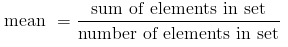Given a set of n elements from a1 to an
a1,a2, a3, a4, .....an-1, an
The mean is found by adding up all the a's and then dividing by the total number, n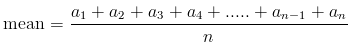This can be generalized by the formula below: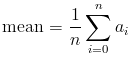Mean Example Problems:-

Example 1: Find the mean of the set of numbers below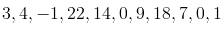Solution:
The first step is to count how many numbers there are in the set, which we shall call n
n = 10
The next step is to add up all the numbers in the set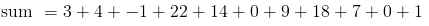sum = 77
The last step is to find the actual mean by dividing the sum by n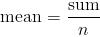mean = 77/10
mean = 7.7
Mean can also be found for grouped data, but before we see an example on that, let us first define frequency.
Frequency in statistics means the same as in everyday use of the word. The frequency an element in a set refers to how many of that element there are in the set. The frequency can be from 0 to as many as possible. If you're told that the frequency an element a is 3, that means that there are 3 as in the set.

Example 2: Find the mean of the set of ages in the table below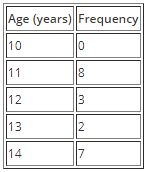Solution:
The first step is to find the total number of ages, which we shall call n. Since it will be tedious to count all the ages, we can find n by adding up the frequencies:
n = 0 + 8 + 3 + 2 + 7
n = 20
Next we need to find the sum of all the ages. We can do this in two ways: we can add up each individual age, which will be a long and tedious process; or we can use the frequency to make things faster.
Since we know that the frequency represents how many of that particular age there are, we can just multiply each age by its frequency, and then add up all these products.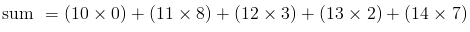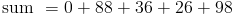sum = 248
The last step is to find the mean by dividing the sum by n
mean = 248/20
mean = 12.4 years

Population Mean vs Sample Mean:-
In the Introduction to Statistics section, we defined a population and a sample whereby a sample is a part of a population.
In statistics there are two kinds of means: population mean and sample mean. A population mean is the true mean of the entire population of the data set while a sample mean is the mean of a small sample of the population. These different means appear frequently in both statistics and probability and should not be confused with each other.
Population mean is represented by the Greek letter μ (pronounced mu) while sample mean is represented by x̄ (pronounced x bar). The total number of elements in a population is represented by N while the number of elements in a sample is represented by n. This leads to an adjustment in the formula we gave above for calculating the mean.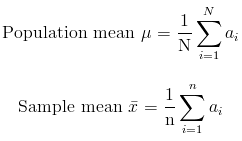The sample mean is commonly used to estimate the population mean when the population mean is unknown. This is because they have the same expected value.

Median:-  The median is defined as the number in the middle of a given set of numbers arranged in order of increasing magnitude. When given a set of numbers, the median is the number positioned in the exact middle of the list when you arrange the numbers from the lowest to the highest. The median is also a measure of average. In higher level statistics, median is used as a measure of dispersion. The median is important because it describes the behavior of the entire set of numbers.

Example 3:- Find the median in the set of numbers given below
15, 16,15, 7,21,18,19,20,11
Solution:
From the definition of median, we should be able to tell that the first step is to rearrange the given set of numbers in order of increasing magnitude, i.e. from the lowest to the highest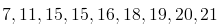Then we inspect the set to find that number which lies in the exact middle.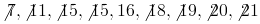median = 16
Lets try another example to emphasize something interesting that often occurs when solving for the median.

Example 4: Find the median of the given data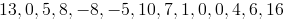Solution:
As in the previous example, we start off by rearranging the data in order from the smallest to the largest.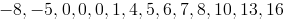Next we inspect the data to find the number that lies in the exact middle.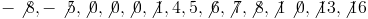We can see from the above that we end up with two numbers (4 and 5) in the middle. We can solve for the median by finding the mean of these two numbers as follows: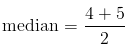median = 9/2
median = 4.5

Mode:-
The mode is defined as the element that appears most frequently in a given set of elements. Using the definition of frequency given above, mode can also be defined as the element with the largest frequency in a given data set.
For a given data set, there can be more than one mode. As long as those elements all have the same frequency and that frequency is the highest, they are all the modal elements of the data set.

Example 5: Find the Mode of the following data set.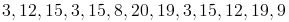Solution: Mode = 3 and 15
Mode for Grouped Data
As we saw in the section on data, grouped data is divided into classes. We have defined mode as the element which has the highest frequency in a given data set. In grouped data, we can find two kinds of mode: the Modal Class, or class with the highest frequency and the mode itself, which we calculate from the modal class using the formula below.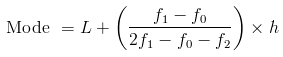where

• L is the lower class limit of the modal class
• f1 is the frequency of the modal class
• f0 is the frequency of the class before the modal class in the frequency table
• f2 is the frequency of the class after the modal class in the frequency table
• h is the class interval of the modal class

Example 6: Find the modal class and the actual mode of the data set below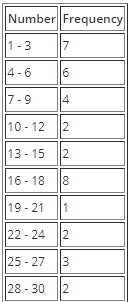Solution: Modal class = 10 - 12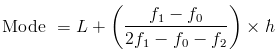where

• L = 10
• f1 = 9
• f0 = 4
• f2 = 2
• h = 3

therefore,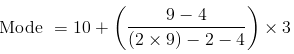Solving the above using the order of operations: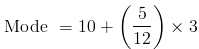Mode = 10 + 1.25
Mode = 11.25

Range:- The range is defined as the difference between the highest and lowest number in a given data set.
Range = Highest Value - Lowest Value

Example 7 : Find the range of the data set below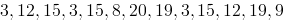Solution: Range = 20 - 3 = 17

Offer running on EduRev: Apply code STAYHOME200 to get INR 200 off on our premium plan EduRev Infinity!

40 docs

,

,

,

,

,

,

,

,

,

,

,

,

,

,

,

,

,

,

,

,

,

;